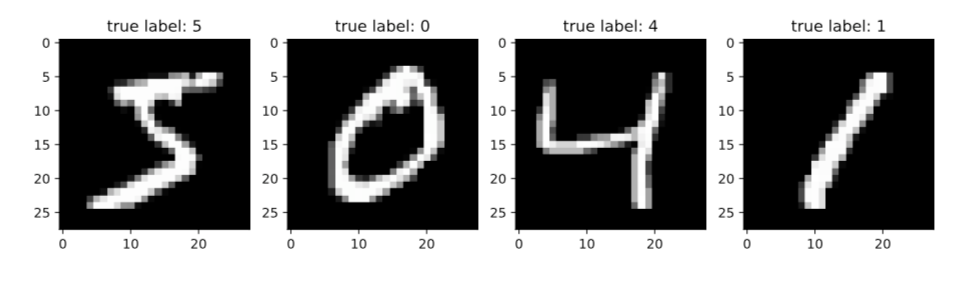# Deep Learning for Image-Like Data

Source: Deep Learning on Medium# Deep Learning for Image-Like Data

From Probabilistic Deep Learning with Python by Oliver Dürr, Beate Sick, and Elvis Murina

This article discusses using deep learning for data that act like images.

__________________________________________________________________

Take 37% off Probabilistic Deep Learning with Python by entering fccdurr into the discount code box at checkout at manning.com.
__________________________________________________________________

## Using a fully connected NN to classify images

Let’s now use your new skills to build a larger network and see how it performs on the task of classifying handwritten digits. Different scientific disciplines have different model systems that benchmark their methods. Molecular biologists use a worm called C. Elegance, people doing social network analysis use the Zachary Karate Club, and finally, people doing DL use the famous MNIST digit data set. This benchmark dataset consists of 70,000 handwritten digits and it’s available from http://yann.lecun.com/exdb/mnist/. The images all have 28 x 28 pixels and are gray scaled with values between 0 and 255. The first four images of the dataset are displayed in Figure 1.

This data set is well-known in the machine learning community. If you develop a novel algorithm for image classification, you usually also report its performance on the MNIST data set. For a fair comparison, there’s a standard split of the data: 60,000 of the images are used for training the network, and 10,000 are used for testing. In Keras, you can download the whole data set with a single line (see listing 3) and also the companion MNIST notebook for this section (on which you can work later) at https://github.com/tensorchiefs/dl_book/blob/master/chapter_02/nb_ch02_02a.ipynb.

Simple neural networks can’t deal with 2D images but need a 1D input vector. Instead of feeding the 28 x 28 images directly, you first flatten the image into a vector of size 28 * 28 = 784. The output should indicate whether the input image is one of the digits zero through nine. More precisely, you want to model the probability that the network thinks that a given input image is a certain digit. For this the output layer has ten neurons (one for each digit). You again use the activation function, softmax, to ensure that the computed outputs can be interpreted as probabilities, which are numbers between zero and one, adding up to one. For this example, we also include hidden layers. Figure 2 shows a simplified version of the network and the definition of the corresponding model in Keras is shown in listing 4.

`from keras.datasets import mnist (x_train, y_train), (x_test, y_test) = mnist.load_data() #A X_train = x_train[0:50000] / 255 #C Y_train = y_train[0:50000] #D Y_train = keras.utils.to_categorical(Y_train,10) #E X_val=x_train[50000:60000] / 255 #F ...`

# A Loads the MNIST training (60,000 images) and test set

# C Uses 50,000 for training and divides by 255; values are in the range 0–1

# D Stores the labels as integers from zero to nine

# F We do the same with the validation set.

Note that we don’t use the testset for this listing.

Also, where we store the labels for the y_train, for the network, we transform those to categorical data of length ten to match the output. A 1 is translated as (0,1,0,0,0,0,0,0,0,0). This is called one-hot encoding.

Listing 4. Definition of an fcNN for the MNIST data

`model = Sequential() model.add(Dense(100, batch_input_shape=(None, 784), #A activation=’sigmoid’)) model.add(Dense(50, activation='’sigmoid’')) #B model.add(Dense(10, activation='softmax')) #C model.compile(loss='categorical_crossentropy', optimizer='adam', # D  metrics=['accuracy']) #D2 history=model.fit(X_train_flat, Y_train,  batch_size=128, epochs=10, validation_data=(X_val_flat, Y_val)  )`

#A The first hidden layer with one hundred neurons, connected to the input size 28*28 pixels

#B A second dense layer with fifty neurons

#C The third layer connecting to the ten output neurons

#D Uses a different optimizer then the SGD, which is faster

#D2 Tracks the accuracy (fraction of correctly classified training and validation examples) during the training